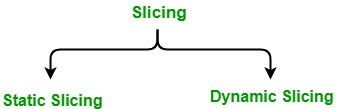# Software Engineering | Slicing

Slicing or program slicing is a technique used in software testing which takes a slice or a group of program statements in the program for testing particular test conditions or cases and that may affect a value at a particular point of interest. It can also be used for the purpose of debugging in order to find the bugs ore easily and quickly.

Slicing techniques were originally defined by Mark Weiser and they were only static in nature at that time. Afterwards, Bogdan Korel and Janusz Laski introduced dynamic slicing, which can work for a particular execution of the program.

It is of 2 types: static slicing and dynamic slicing, these are explained as following below:1. Static slicing:

• A static slice of a program contains all statements that may affect the value of a variable at any point for any arbitrary execution of the program.
• Static slices are generally larger.
• It considers every possible execution of the program.

2. Dynamic slicing:

• A dynamic slice of a program contains all the statements that actually affect the value of a variable at any point for a particular execution of the program.
• Dynamic slices are generally smaller.
• Considers only a particular execution of the program.

Let us see an example by the given code snippet:

 `int` `z = 10; ` `int` `n; ` `cin >> n; ` `int` `sum = 0; ` `if` `(n > 10) ` `    ``sum = sum + n; ` `else` `    ``sum = sum - n; ` `cout << ``"Hey"``; `

Static slice for the variable sum:

 `int` `n; ` `cin >> n; ` `int` `sum = 0; ` `if` `(n > 10) ` `    ``sum = sum + n; ` `else` `    ``sum = sum - n; `

Dynamic slice for the variable sum when n = 22;

 `int` `n; ` `cin >> n; ` `int` `sum = 0; ` `if` `(n > 10) ` `    ``sum = sum + n; `

As it can be observed in the above example that static slice takes all the possible execution (in this case it is 2) of the program which may affect the value of the variable sum. Whereas in case of dynamic slicing, it considers only a particular execution (when n = 22) of the program which may affect the value of the variable sum.
Hence, dynamic slice is always smaller than a static slice.

My Personal Notes arrow_drop_upCheck out this Author's contributed articles.

If you like GeeksforGeeks and would like to contribute, you can also write an article using contribute.geeksforgeeks.org or mail your article to contribute@geeksforgeeks.org. See your article appearing on the GeeksforGeeks main page and help other Geeks.

Please Improve this article if you find anything incorrect by clicking on the "Improve Article" button below.

Article Tags :

Be the First to upvote.

Please write to us at contribute@geeksforgeeks.org to report any issue with the above content.